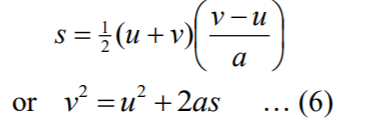Equations of Motion﻿⁕ Kinematic Equations of Motion (Graphical Treatment)

↪ For an object moving in a straight line the displacement, velocity, uniform acceleration and time are related by some equations which are known as kinematic equations of motion.↪ Suppose the velocity of an object moving with uniform acceleration increases from the initial value u to final value v in time t.
↪ The v-t graph is a straight line PQ such that
OP = u,
MQ = v,
OM = t
and RQ = MQ – MR = MQ – OP = v – u

↪ It is known that the acceleration of the object is equal to the slope of the v-t graph.

∴ acceleration = slope of PQ↪ The displacement of the object at any time t is equal to the area of trapezoid OPQM in the v-t graph.
↪ It is known that

displacement = area of trapezoid OPQM↪ From (3), putting the value of v in (4), we get↪ From (2), putting the value of t in (4), we get↪ Therefore we have four kinematic equations such as↪ For an object traveling with a uniform velocity, u = v, and a = 0.
↪ The four equations reduce to a single equation s = ut.

↪ For an object falling vertically downward under action of gravity, a = g and s = h where g is the acceleration due to gravity and h is the vertical height through which the object is displaced.↪ For an object rising vertically upward against action of gravity, a = – g and s = h⁕ Distance Traveled by Uniformly Accelerated Object in a Particular Second

↪ Suppose an object has an initial velocity u and uniform acceleration a.

↪ The distance traveled in n seconds is given byand the distance traveled in (n – 1) seconds is given by↪ So the distance traveled by the object in nth second is↪ This is the distance traveled by the uniformly accelerated object in nth second.